ShuffleNet-v2简介与实现• 累计撰写 313 篇文章
• 累计收到 50 条评论

# ShuffleNet-v2简介与实现2021-11-27 / 0 评论 / 1,050 阅读 / 正在检测是否收录...

## 1.原论文

ECCV2018--ShuffleNet V2: Practical Guidelines for Efficient CNN Architecture Design

## 2.设计理念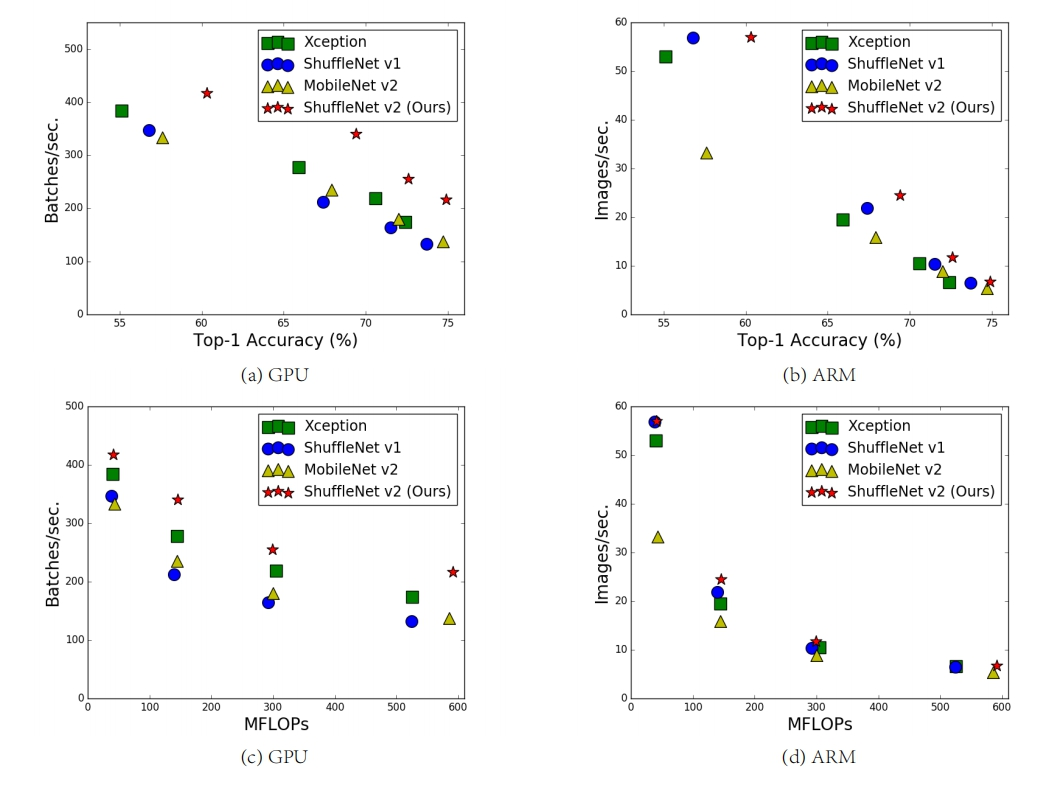## 3.根据设计理念及实验得出的4条基本设计准则

### 3.1探索实验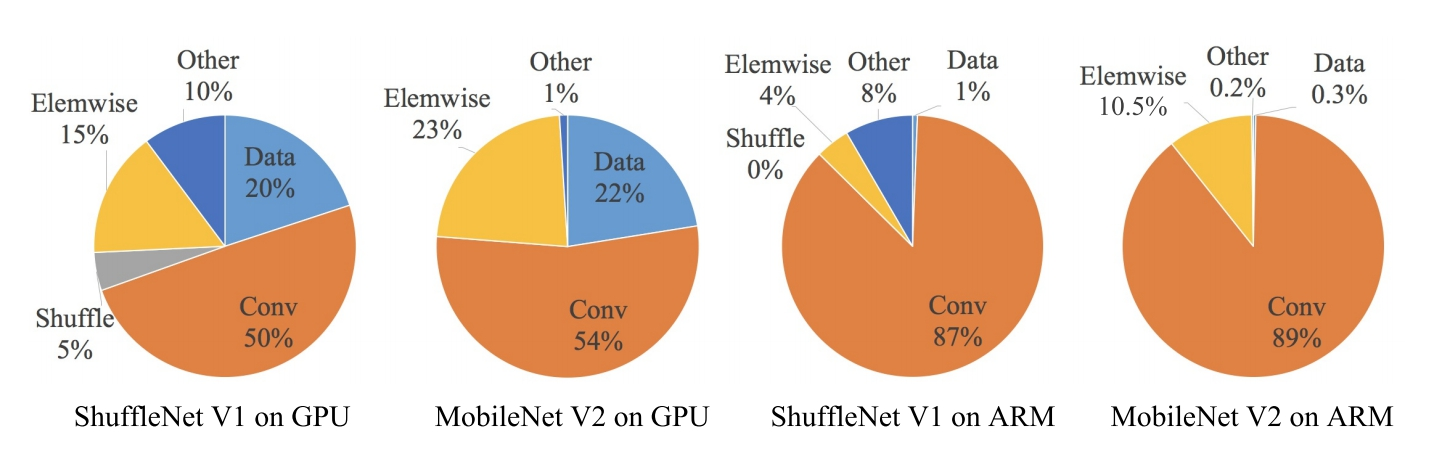#### G1:同等通道大小最小化内存访问量

$$B=hwc_1c_2$$

$$MAC = hw(c_1+c_2)+c_1c_2$$

$$MAC \ge 2 \sqrt{hwB} + \frac{B}{hw}$$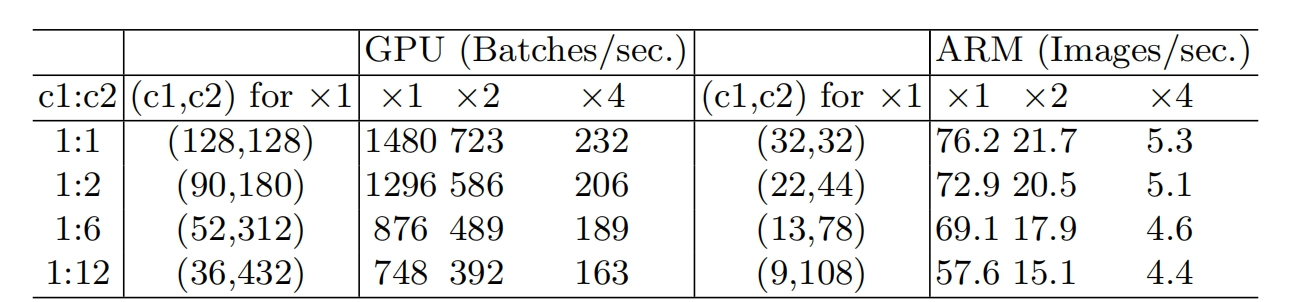#### G2:过量使用组卷积会增加MAC(memory access cost)

$$B = hwc_1c_2/g$$

$$MAC = hwc_1+Bg/c_1+B/hw$$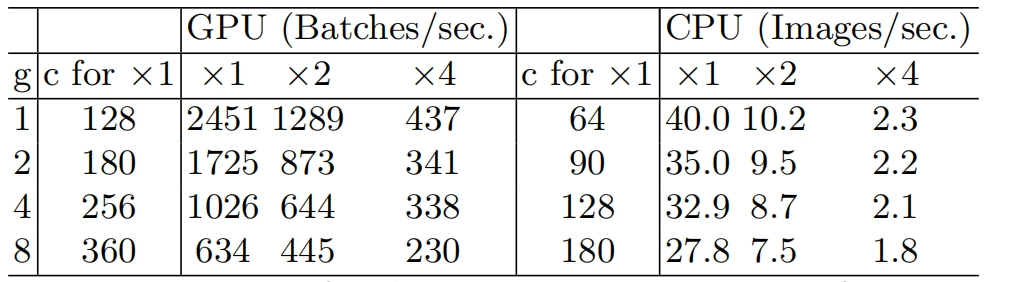#### G3:网络碎片化会降低并行度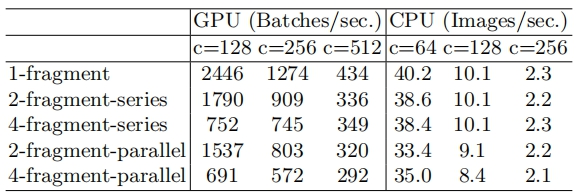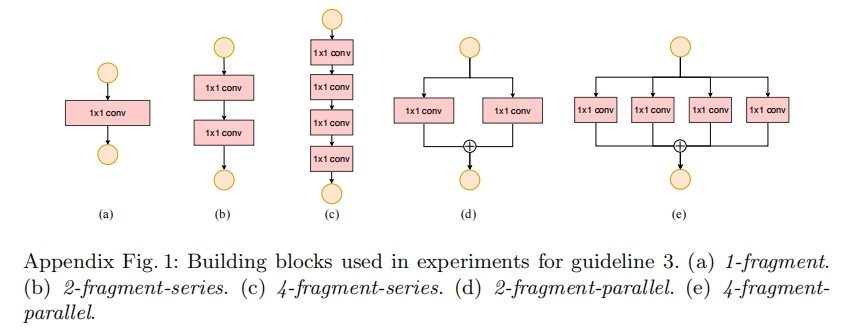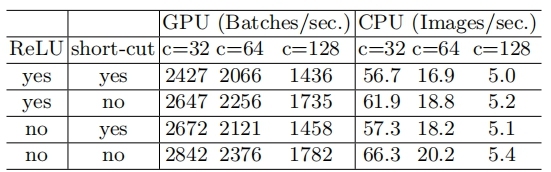## 4.ShuffleNet-v1 基本结构及存在的问题

### 4.1ShuffleNet-v1基本结构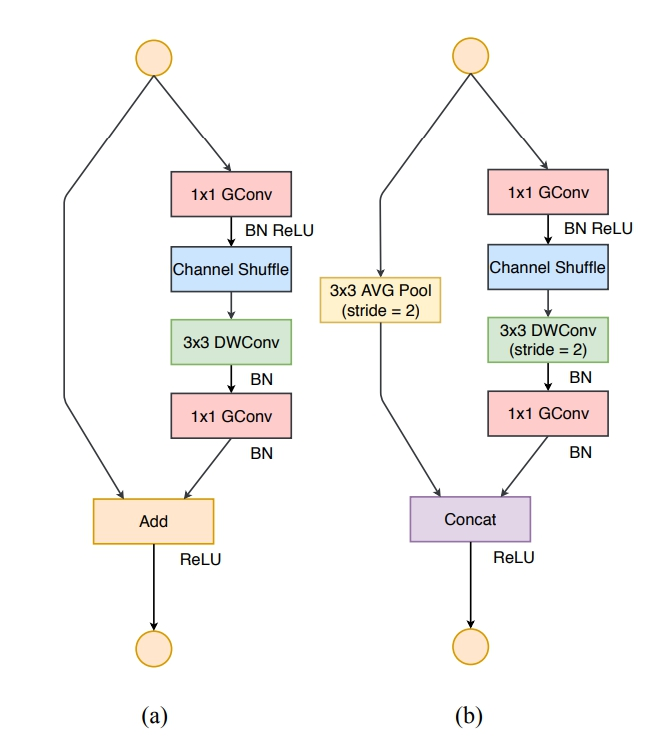• (a) the basic ShufflleNet unit;
• (b) the ShufflleNet unit for spatial down sampling (2×);

### 4.2ShuffleNet-v1存在的问题

• 在ShuffleNetv1的模块中，大量使用了1x1组卷积，这违背了G2原则，
• 另外v1采用了类似ResNet中的瓶颈层（bottleneck layer），输入和输出通道数不同，这违背了G1原则。
• 同时使用过多的组，也违背了G3原则。

## 5.ShuffleNet-v2基本块及改进分析

### 5.1ShuffleNet-v2基本块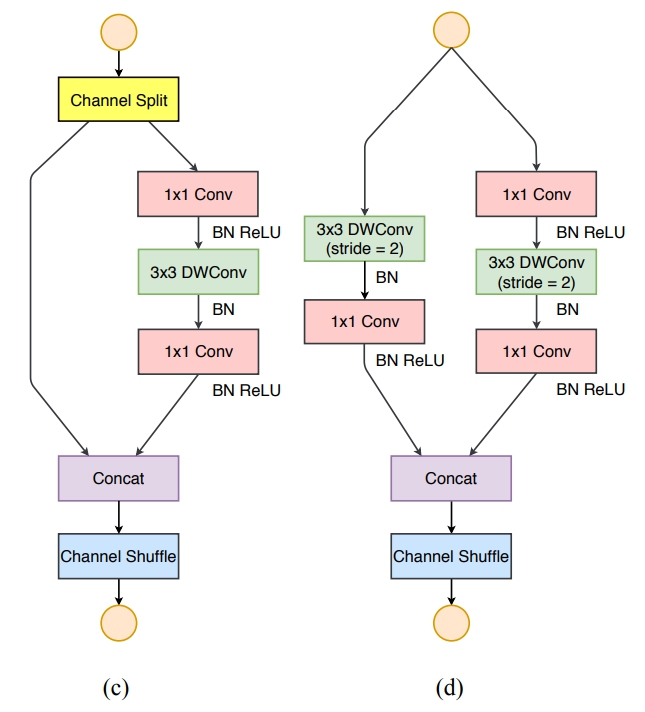• (c) the basic ShufflleNetv2 unit;
• (d) the ShufflleNetv2 unit for spatial down sampling (2×);
• DWConv: depthwise convolution
• GConv:group convolution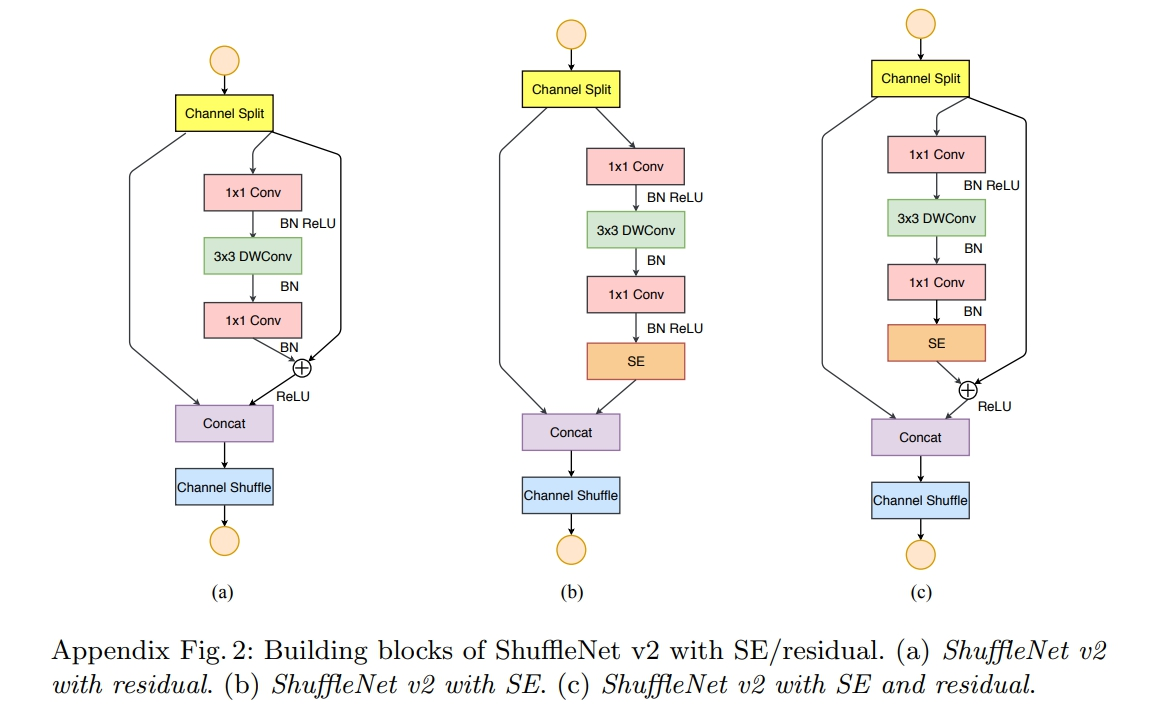### 5.2ShuffleNetv2的改进分析

channel split的做法：

• 在开始时先将输入特征图在通道维度分成两个分支：通道数分别为 $c'$ 和 $c-c'$ ，实际实现时$c'=c/2$ 。
• 一个是identity，一个经过三个conv，然后concat到一起，这个满足G4。
• 右边的分支包含3个连续的卷积，并且输入和输出通道相同，这符合G1
• 而且两个1x1卷积不再是组卷积，这符合G2

channel split的作用：

• 第一，划分一半到右分支，意味着右边计算量减少，从而可以提高channel数，提高网络capacity。
• 第二，左分支相当于一种特征重用(feature reuse), 跟DenseNet和CondenseNet一样的思想。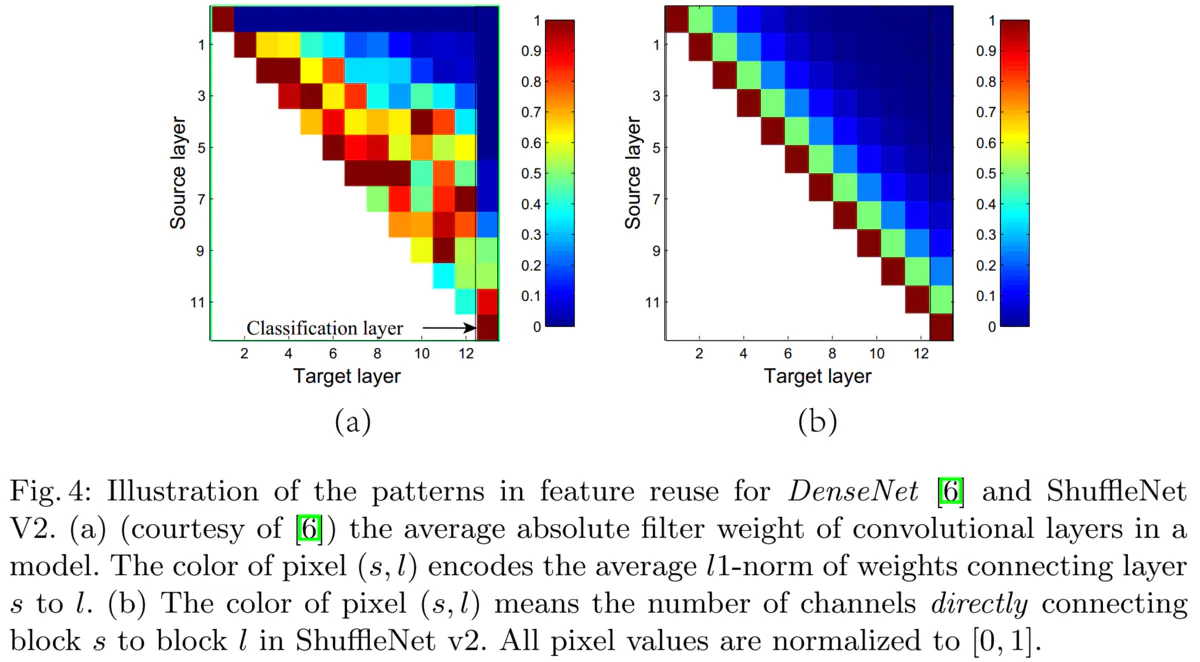## 6.ShuffleNet-v2完整网络结构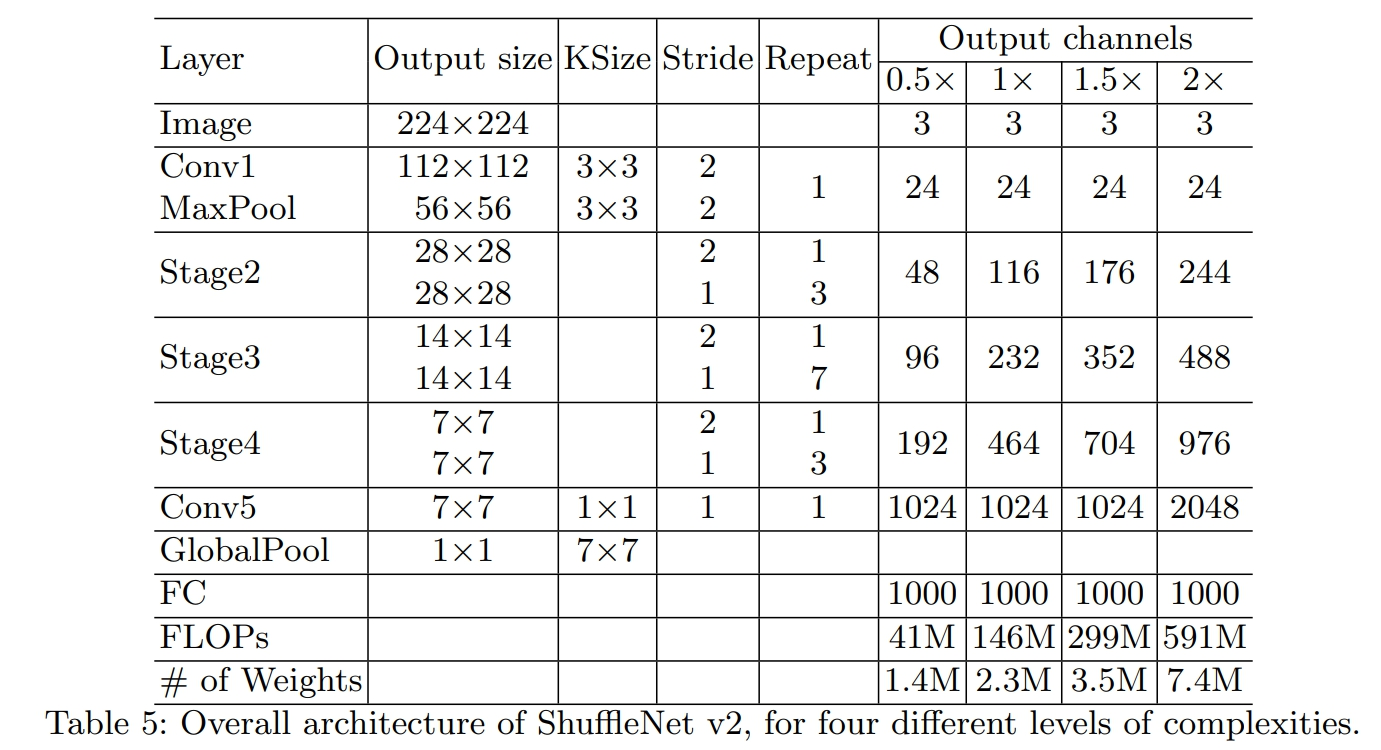• 1个stage=1个DSampling+Repeat个BasicUnit

## 7.ShuffleNet-v2基本块实现(pytorch)

### 7.1Channel Shuffle

• 图示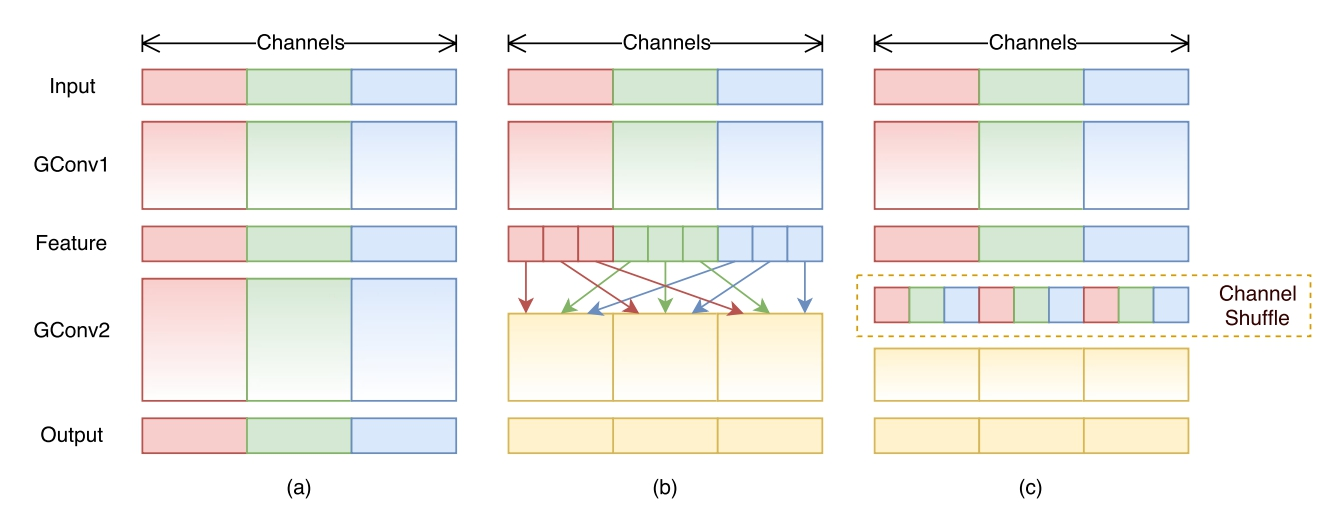• 实现步骤

• 假定将输入层分为 g 组，总通道数为 g × n 。
• 首先你将通道那个维度拆分为 (g,n) 两个维度
• 然后将这两个维度转置变成 (n,g)
• 最后重新reshape成一个维度 g × n 。
• 代码
#  Channel Shuffle
def shuffle_chnls(x, groups=2):
"""Channel Shuffle"""

bs, chnls, h, w = x.data.size()

# 如果通道数不是分组的整数被，则无法进行Channel Shuffle操作，直接返回x
if chnls % groups:
return x

# 计算用于Channel Shuffle的一个group的的通道数
chnls_per_group = chnls // groups

# 执行channel shuffle操作
x = x.view(bs, groups, chnls_per_group, h, w) # 将通道那个维度拆分为 (g,n) 两个维度
x = torch.transpose(x, 1, 2).contiguous() # 将这两个维度转置变成 (n,g)
x = x.view(bs, -1, h, w) # 最后重新reshape成一个维度 g × n g\times ng×n

return x

### 7.2ShufflleNetv2基本块( the basic ShufflleNetv2 unit)

• 基本结构图示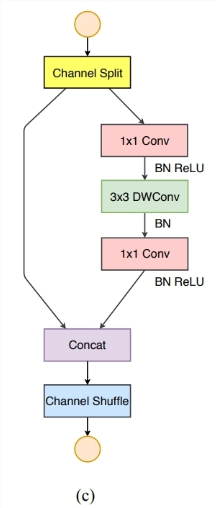• 增加SE(Squeeze-and-Excitation)模块和残差(residual)结构后的基本块结构图示• 代码
#  封装一个Conv+BN+RELU的基本块
class BN_Conv2d(nn.Module):
def __init__(self, in_channels, out_channels, kernel_size, stride, padding,
dilation=1, groups=1, bias=False, activation=True): # dilation=1-->卷积核膨胀
super(BN_Conv2d, self).__init__()
layers = [nn.Conv2d(in_channels, out_channels, kernel_size=kernel_size, stride=stride,
nn.BatchNorm2d(out_channels)]
if activation:
layers.append(nn.ReLU(inplace=True))
self.seq = nn.Sequential(*layers)

def forward(self, x):
return self.seq(x)
# ShuffleNet-v2基本块
class BasicUnit(nn.Module):
def __init__(self, in_chnls, out_chnls, is_se=False, is_residual=False, c_ratio=0.5, groups=2):
super(BasicUnit, self).__init__()
self.is_se, self.is_res = is_se, is_residual # 是否使用SE结构和残差结构
self.l_chnls = int(in_chnls * c_ratio) # 左侧输入通道数
self.r_chnls = in_chnls - self.l_chnls # 右侧输入通道数
self.ro_chnls = out_chnls - self.l_chnls # 右侧输出通道数
self.groups = groups

# layers
self.conv1 = BN_Conv2d(self.r_chnls, self.ro_chnls, 1, 1, 0)
self.dwconv2 = BN_Conv2d(self.ro_chnls, self.ro_chnls, 3, 1, 1,  # same padding, depthwise conv
groups=self.ro_chnls, activation=None)
act = False if self.is_res else True
self.conv3 = BN_Conv2d(self.ro_chnls, self.ro_chnls, 1, 1, 0, activation=act)

# 是否使用SE模块和residual结构
if self.is_se:
self.se = SE(self.ro_chnls, 16)
if self.is_res:
self.shortcut = nn.Sequential()
if self.r_chnls != self.ro_chnls:
self.shortcut = BN_Conv2d(self.r_chnls, self.ro_chnls, 1, 1, 0, activation=False)

def forward(self, x):
# channel split 操作
x_l = x[:, :self.l_chnls, :, :]
x_r = x[:, self.r_chnls:, :, :]

# right path
out_r = self.conv1(x_r)
out_r = self.dwconv2(out_r)
out_r = self.conv3(out_r)

# 是否使用SE模块和residual结构
if self.is_se:
coefficient = self.se(out_r)
out_r *= coefficient
if self.is_res:
out_r += self.shortcut(x_r)

# concatenate
out = torch.cat((x_l, out_r), 1)
return shuffle_chnls(out, self.groups)

### 7.3ShufflleNetv2下采样基本块(the ShufflleNetv2 unit for spatial down sampling)

• 图示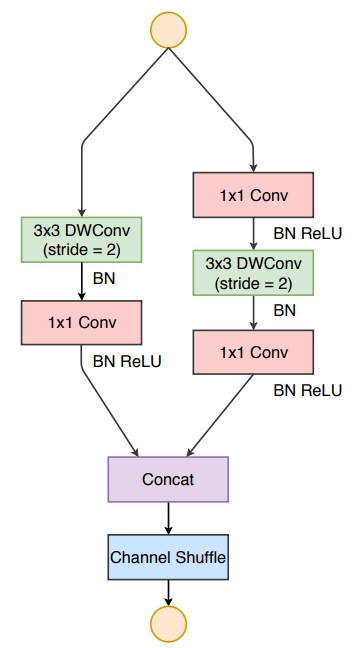• 代码
# SuffleNet-v2下采样基本块
class DSampling(nn.Module):
def __init__(self, in_chnls, groups=2):
super(DSampling, self).__init__()
self.groups = groups
# down-sampling(通过stride=2实现), depth-wise conv(通过groups=in_chnls实现).
self.dwconv_l1 = BN_Conv2d(in_chnls, in_chnls, 3, 2, 1,
groups=in_chnls, activation=None)
self.conv_l2 = BN_Conv2d(in_chnls, in_chnls, 1, 1, 0)
self.conv_r1 = BN_Conv2d(in_chnls, in_chnls, 1, 1, 0)
self.dwconv_r2 = BN_Conv2d(in_chnls, in_chnls, 3, 2, 1, groups=in_chnls, activation=False)
self.conv_r3 = BN_Conv2d(in_chnls, in_chnls, 1, 1, 0)

def forward(self, x):
# left path
out_l = self.dwconv_l1(x)
out_l = self.conv_l2(out_l)

# right path
out_r = self.conv_r1(x)
out_r = self.dwconv_r2(out_r)
out_r = self.conv_r3(out_r)

# concatenate
out = torch.cat((out_l, out_r), 1)
return shuffle_chnls(out, self.groups)

## 8.ShuffleNet-v2网络结构实现

# TODO

0